#### Thank you for registering.

One of our academic counsellors will contact you within 1 working day.

Please check your email for login details.

Click to Chat

1800-1023-196

+91-120-4616500

CART 0

• 0
MY CART (5)

Use Coupon: CART20 and get 20% off on all online Study Material

ITEM
DETAILS
MRP
DISCOUNT
FINAL PRICE
Total Price: Rs.

There are no items in this cart.
Continue Shopping# Area under CurvesThe most important topic of Integral calculus is Calculation of area. Integration in general is considered to be a tough topic and area calculation tests a person’s Integration and that too definite integral which is all the more difficult. Integration including both Definite and Indefinite integrals lays the groundwork for the questions of area calculation in Integral calculus.

In this chapter we have basically discussed the basic concepts of area under curves followed by the working rule of finding the area. The concepts have been explained in detail along with supportive illustrations wherever required. We have also included ‘the concept of definite integration and its application for calculation of area of the regions bounded by specific curves. We have tried to cover all the major types of questions which are likely to be encountered by students in various competitive exams.

Area Calculation is important from the perspective of scoring high in IIT JEE as there are few fixed patterns on which a number of Multiple Choice Questions are framed. You are expected to do all the questions based on this to remain competitive in IIT JEE examination.

Watch this Video for more reference

We have learnt that the definite integral between two values of Independent variable represents the area of the curve bound by the curve, the axis of the independent variable. Further, as we can calculate the area under one curve and the area under another curve then we can calculate the area between two curves. Depending upon the nature of the curves, this area can have different shapes and thus the tool of definite integral can be employed to calculate the area of different shapes. As a matter of fact, you will realize that the standard formulae to calculate the areas of different shapes can be derived by definite integral by choosing the appropriate curves.

Various sub heads included under this topic are listed below:

Generally speaking, when we aim at calculating the area bounded by a curve, we have a figure of the type given below:Here, the area ‘S’ covered by the curve f(x) is the area we wish to calculate. The area ‘S’ covered by the curve f(x) between x = a and x = b can be found by integrating the curve f(x) from a to b.

### Some of the basic points to be kept in mind while dealing with the questions of this topic are:

• A graph is of utmost importance in these questions. The bounding region provides the limits of integration and it is not easy to do that without a graph.

• It is very confusing to determine which function is an upper function and which is lower without a graph. So in order to avoid any mistake, students are advised to first draw a graph to the question so as to have a clear picture of what exactly is being asked.

• The area between the graph y= f(x) and the x-axis is given by the definite integral as given below. This formula gives a positive answer for a graph above the x-axis and a negative answer for the one below the x- axis. In case, the graph is partly below and partly above the x-axis, the formula gives the net resultant area i.e. the area above minus the area below the x-axis.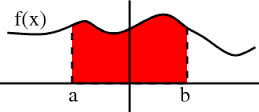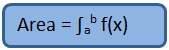Before we try attempting questions on area under curves, it is important to have an idea about the concepts related to curves. We first throw some light on such concepts:
• ### Asymptotes:

Asymptotes are the lines whose distance from the curve tends to zero as the point on the curve moves towards infinity along the branch of the curve.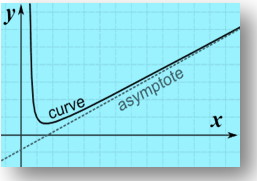• ### Conditions for various asymptotes:

1. If lim x→a f(x) = ∞ or lim x→a f(x) = -∞, then x = a is an asymptote of y = f(x).

2. If lim x→ +∞ f(x) = k or lim x→ -∞ f(x) = k, then y = k is an asymptote of y = f(x).

3. If lim x→-∞ f(x)/x = a2 and lim x→-∞ (f(x) – a2x) = c*, then y = a2x + c* is an asymptote which is inclined towards the left.

4. If lim x→∞ f(x)/x = a1 and lim x→∞ (f(x) – a1x) = c, then y = a1x + c is an asymptote which is inclined towards the right.

A student needs to be really proficient in curve tracing as if one is able to draw the curve of the function, then it becomes quite simple to find the required area. We discuss here some of the ways which can help a student in judging the type of curve:

1. If all the powers of y in the equation of curve are even, then the curve is symmetrical about the x-axis. For eg: y2 = 4ax1. Similarly, if all the powers of y in the equation of curve are even, then the curve is symmetrical about the y-axis. For eg: x2 = 4ay1. If all the powers of x and y in the equation of the curve are even, then the curve is symmetrical both about x as well as y-axis. Eg: x2 + y2 = a2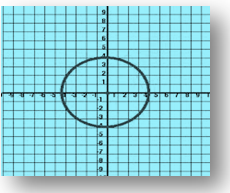1. If the equation of the curve remains unchanged on interchanging x and y, then the curve is symmetrical about the line y = x.1. If the equation of the curve remains unaltered when x and y are replaced by their negatives i.e. –x and –y respectively, the curve is symmetric in opposite quadrants. Eg: xy = c2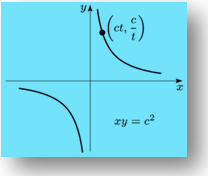Various working rules for calculation of area have been discussed in the coming sections. Thus, students wanting to get an in-depth knowledge of area under curves can refer the later sections.

### Some Key Facts:

• The curve which lies completely above the x-axis is obviously positive while if it lies below the x-axis then the area is negative.

• By convention, only the magnitude of the area is taken into consideration.

• If the curve lies completely above or below the x-axis then it can be easily decided whether it will be positive or negative. But, at times, the curve may lie partly above the x-axis and partly below the x-axis. In that case, both the areas must be computed separately. The resultant area is then computed by adding up their moduli.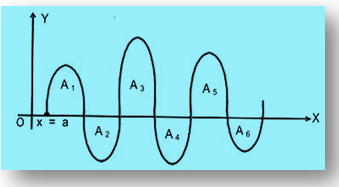• In case, the curve y = f(x) crosses the x-axis around n times when x varies from a to b, then the areas between y = f(x), the x-axis and the lines x = a and x = b is given by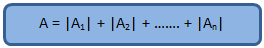• If the curve is symmetrical about the x-axis, or the y-axis, or both, then instead of computing the entire area, just the area of one of the symmetrical part can be found out calculate the area of one symmetrical part and multiply it by the number of symmetrical parts to get the whole area.

• Let f(x) be a continuous function in (a, b). Then the area bounded by the curve

1.  y = f(x), the x-axis and the lines x = a and x = b is given by the formulae A = | ∫ab f(x) dx|, provided f(x) > 0 (or f(x) < 0) ∀ x ∈ (a, b).

2. The area bounded by x = f(y), the y-axis and the lines y = c and y = d is given by A = | ∫cd f(y) dy|, provided f(y) > 0 or f(y) < 0 ∀ y ∈ (c, d).

3. If we have two functions f(x) and g(x) such that f(x) < g(x) ∀ x ∈ [a, b], then the area bounded by the curves y = f(x), y = g(x) and the lines x = a, x = b (a < b) is given by A =| ∫ab [g(x) – f(x)] dx|.

### Illustration 1:

Determine the area of the region bounded by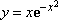,y=x+1, x=2 and the y-axis.

### Solution:

Firstly, as explained above, draw the figure so as to have a clear picture. The corresponding graph is given below:Hence, it is clear from the figure that area of the shaded portion is given by:### Illustration 2:

A curve passes through (2, 0) and the slope of tangent at point P(x, y) equals [(x+1)2 + y – 3]/(x +1). Find the equation of curve and the area enclosed by the curve and the x-axis in the fourth quadrant.(IIT JEE 2004)

### Solution:

The slope of the tangent is given as [(x+1)2 + y – 3]/(x +1).

This means that dy/dx = [(x+1)2 + y – 3]/(x +1)

SO, dy/dx = (x+1) + (y-3)/(x+1)

Put x + 1 = X and y – 3 = Y

⇒ dy/dx = dY/dX

Hence, dY/dX = X + Y/X

This gives dY/dX – 1/X Y = X

Now, integrating factor, I.F = exp(-1/X)dx = e -log X = 1/X

Hence, the solution is Y.1/X = ∫X.1/X dX + c

This gives Y/X = X + c

y – 3 = (x +1)2 + c(x + 1)Since this passes through (2, 0) so, -3 = (3)2 + 3c

This yields c = -4.

Hence, the required curve is y = (x+1)2 – 4(x+1) + 3

y = x2 – 2x

Hence, the required area is given by,

A =

= 8/3 – 4

= 4/3 sq unit.1. A curve remains unchanged on interchanging x and y, then the curve is symmetrical about

(a) x axis                                  (b) y = x                                      (c) y axis                              (d) None of these

1. Area enclosed by the curve |x| + |y| = 1 is

(a) 2                                        (b) 3                                           (c) √2                                    (d) 1

1. a curve lies partly above the x-axis and partly below it. The area of the curve can be

(a) only positive                       (b) only negative                        (c) May be positive or negative (d) None of these

Answers:  1(b), 2(a), 3(c).

## Related Resources

To read more, Buy study materials of Application of Integration comprising study notes, revision notes, video lectures, previous year solved questions etc. Also browse for more study materials on Mathematics here.## Calculus

OFFERED PRICE: Rs. 1,696
View Details Xpress Buy

### Course Features

• 51 Video Lectures
• Revision Notes
• Test paper with Video Solution
• Mind Map
• Study Planner
• NCERT Solutions
• Discussion Forum
• Previous Year Papers

### Course Features

• 731 Video Lectures
• Revision Notes
• Previous Year Papers
• Mind Map
• Study Planner
• NCERT Solutions
• Discussion Forum
• Test paper with Video Solution